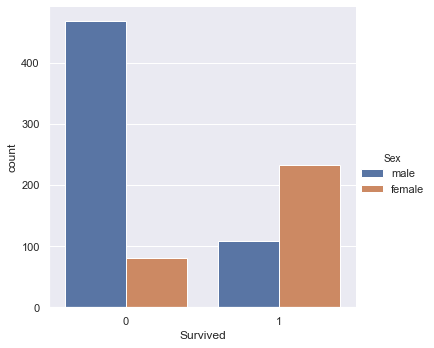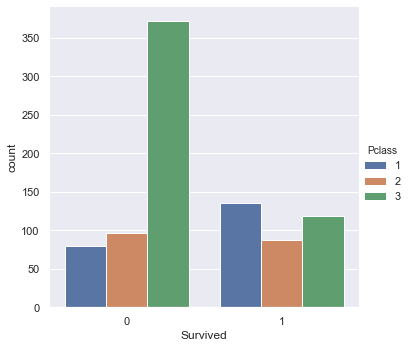In :
import pandas as pd


# 과제1¶

In :
survived_male = train.loc[(train['Sex'] == 'male') & (train['Survived'] == 1), 'PassengerId'].count()
survived_female = train.loc[(train['Sex'] == 'female') & (train['Survived'] == 1), 'PassengerId'].count()
dead_male = train.loc[(train['Sex'] == 'male') & (train['Survived'] == 0), 'PassengerId'].count()
dead_female = train.loc[(train['Sex'] == 'female') & (train['Survived'] == 0), 'PassengerId'].count()

df

Out:
male female
Survived 109 233

# 과제2¶

In :
%matplotlib inline

import matplotlib.pyplot as plt
import seaborn as sns
sns.set()

In :
sns.catplot(data=train, x='Survived', hue='Sex', kind='count')

Out:
<seaborn.axisgrid.FacetGrid at 0x1d5fe87f0c8>In :
sns.catplot(data=train, x='Survived', hue='Pclass', kind='count')

Out:
<seaborn.axisgrid.FacetGrid at 0x1d5fcde9e48>In [ ]: